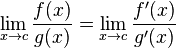# A problem with limits ( i think)

• steve2510

## Homework Statement

The maximum deflection of a beam is given by the equation
y=M/P(sec(μL/2)-1)
μ=√(P/EI) Where EI Is a constant.
Show that as P→0 y→ML^2/8EI

This is a mathCAD problem by the way, but I'm very novice at it so i want to try formulise some sort of solution on paper before i attempt mathCAD

## Homework Equations## The Attempt at a Solution

I'm really stuck, firstly i would replace μ with √(P/EI)
∴y = M/P(sec(√(P/EI)*L)/2)-1)
I think the next step is to try get the EI out the function as it is a constant and doesn't need to be differntiated.
The derivivative of secx is tanxsecx which is the same as tanx/cosx and as x approaches 0 the function will equal 0/1 which is 0

If someone could point me in the right direction it would be much appreciated !

The limit is correct, if the initial equation has P^2 instead of P in the denominator.

I would keep EI there, it gives the correct factors in the derivatives.
$$y=M \frac{\sec \left(\sqrt{\frac{P}{EI}}\frac{L}{2} \right)-1}{P^2}$$

So you want to replace $\sqrt{P/EI}L/2$ with x. Then $P= (4x/L^2)(EI)= 4EIx/L^2$. $y= M/[(4EIx/L^2)(sec(x)- 1)= ML^2/(4EIx(sec(x)- 1)$

I'm still a little bit confused have you said that the √x = √P/EI * L/2 ∴ x = P/EI * L^2/4 ∴ 4xEI/L^2 = P, then

I follow that you have placed P back into the equation I am confused why the sec x is on the bottom of the fraction now when it was on the top before.

Am i not right in saying that y=(ML^2*sec(x)-1)/4EIx
So d/dx(Ml^2*sec(x)-1) = ML^2secxtanx
d/dx 4EIx = 4Ei
Would i then subsitute x back into the equation ?

Also Mfb why have you replaced P with P^2 in the original equation

I think some brackets in HallsofIvy's post are wrong.
Also Mfb why have you replaced P with P^2 in the original equation
That is just a guess: with P^2, the given limit is correct, without, it is not.

So I've said that x = √(P/Ei) * L/2
∴P = (x2*EI *4)/L2

Subsituting back in i get

(ML2(sec(x)-1)/x24EI

D/dx ML2(sec(x)-1) = ML2sec(x)tan(x) Where x = √(P/Ei) * L/2 as P reaches 0 x = 0 aswell. Which means sec(x)tan(x) = zero which cancells the top complety so surely this isn't correct!?

The bottom would become 8EIx

Am i doing something wrong, this is really my first problem with limits and i don't even recall being taught L'Hôpital's rule. This is a MathCad problem, and i think it is meant to calculate mos this maths!

I'm just sturggling to arrange it in mathCAD without getting a catalogue of errors, so i thought it might be easier to generate a paper solution first !

I still don't see where the p^2 came from , if p is approaching 0 then what difference does it make if p is p^2, also if its p^2 on the bottom would you not have to times the top by another factor of p. whether p is p^2 or just p surely we have the correct intermediate form of 0/0 already as secx -1 = 0

I still don't see where the p^2 came from , if p is approaching 0 then what difference does it make if p is p^2, also if its p^2 on the bottom would you not have to times the top by another factor of p. whether p is p^2 or just p surely we have the correct intermediate form of 0/0 already as secx -1 = 0
That P^2 is not the result of any calculation.

The formula given in post 1 does not fit to the limit given there. Therefore, we have 3 options:
- the given limit is wrong. In that case, you already know the correct limit
- the given formula is wrong, but the limit is right. In that case, I found a new, different formula, with the correct limit
- both are wrong. We are lost.